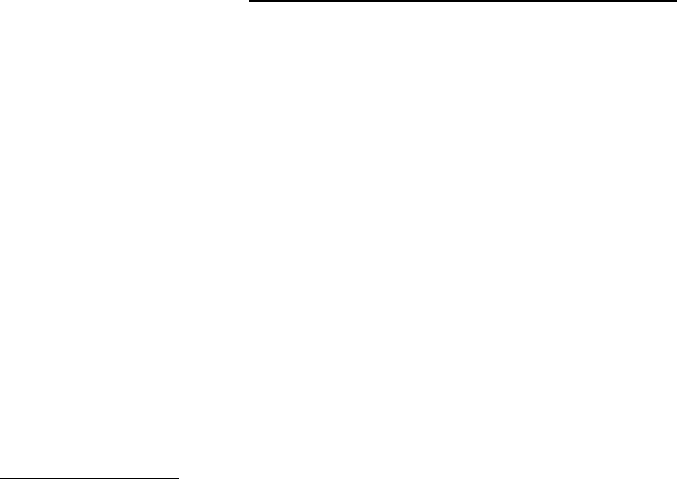Textbook Notes (290,000)
CA (170,000)
York (10,000)
OMIS (50)
Chapter 17

# OMIS 2010 Chapter Notes - Chapter 17: Response Surface Methodology, Linear Regression, F-Test

Department
Operations Management and Information System
Course Code
OMIS 2010
Professor
Alan Marshall
Chapter
17

This preview shows page 1. to view the full 4 pages of the document.Chapter 17: Multiple Regression
Model and Required Conditions
-Assume that “k” independent variables potentially related to dependent variable
-Y = B0 + B1x1 + B2x2…..+ Bkxk +e
oY is the dependent variable
oX1, x2….. xk are independent variables
oE is error variable
oB0, B1…. Bk are coefficients
-Deviations between additional independent variables included, deviations between predicted values
of y” and actual values of “y” will occur
-Response surface: graphical depiction of equation when more than independent variable is in regression
model
Required Conditions for Error Variable
-Probability distribution of the error variable is normal
-Mean of error variable is 0
-Standard deviation of error variable is a constant
-Errors are independent
Estimating Coefficients and Assessing the Model
-Determine how well model fits the data
-If fit is poor then no point in further analysis of coefficients of that model
1) Select variables that you think are linearly related to dependent variable
2) Generate coefficients and stats used to assess the model
3) Diagnose violations of required conditions
4) Assess models fit; use standard error of estimate, coefficient of determination and F-test of
ANOVA
5) Interpret the coefficient; use the model to predict value of dependent variable or estimate expected
value of dependent variable
-Only screen independent variables and include those that affect dependent variable